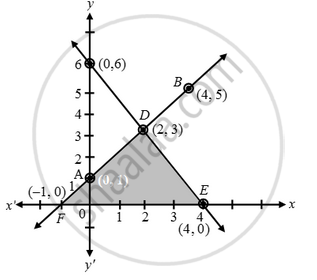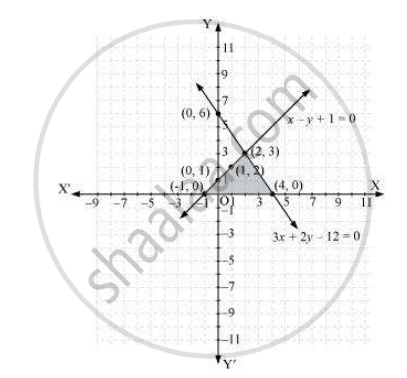# Draw the graphs of the equations x – y + 1 = 0 and 3x + 2y – 12 = 0. Determine the coordinates of the vertices of the triangle formed by these lines and the x-axis, and shade the triangular region. - Mathematics

Draw the graphs of the equations x – y + 1 = 0 and 3x + 2y – 12 = 0. Determine the coordinates of the vertices of the triangle formed by these lines and the x-axis, and shade the triangular region.

#### Solution 1

Pair of linear equations are:

x – y + 1 = 0 ….(1)

3x + 2y – 12 = 0 ….(2)

x – y + 1 = 0 ⇒ y = x + 1

 x 0 4 y 1 5 Points A B

3x + 2y – 12 = 0 ⇒ y = \frac { 12-3x }{ 2 }

 x 0 2 y 6 3 Points C D

Plot the points A(0, 1), B(4, 5) and join them to get a line AB. Similarly, plot the points C(0, 6), D(2, 3) and join them to form a line CD.Clearly, the two lines intersect each other at the point D(2, 3). Hence x = 2 and y = 3 is the solution of the given pair of equations.

The line CD cuts the x-axis at the point E (4, 0) and the line AB cuts the x-axis at the point F(–1, 0).

Hence, the coordinates of the vertices of the triangle are; D(2, 3), E(4, 0), F(–1, 0).

Verification:
Both the equations (1) and (2) are satisfied by x = 2 and y = 3. Hence, Verified.

#### Solution 2

x - y + 1 = 0

x = y - 1

 x 0 1 2 y 1 2 3

3x + 2y - 12 = 0

x = (12 - 2y)/3

 x 4 2 0 y 0 3 6

Hence, the graphic representation is as follows.From the figure, it can be observed that these lines are intersecting each other at point (2, 3) and x-axis at ( - 1, 0) and (4, 0). Therefore, the vertices of the triangle are (2, 3), ( - 1, 0), and (4, 0).

Concept: Graphical Method of Solution of a Pair of Linear Equations
Is there an error in this question or solution?

#### APPEARS IN

NCERT Class 10 Maths
Chapter 3 Pair of Linear Equations in Two Variables
Exercise 3.2 | Q 7 | Page 50